# tkinter的选项卡组件Notebook详细讲解

参数：

height : 组件高度

width : 组件宽度

参数：

text : 选项卡的字符串名称

state : 选项：normal,  disabled（禁止）,  hidden（隐藏）

image : 选项卡插入图片

compound ：选项卡上同时显示图片和文字时，彼此之间的位置关系

```from tkinter import *
from tkinter.ttk import *

root=Tk()
root.geometry('300x150+888+444')

note1=Notebook(root) # 1 创建Notebook组件
note1.pack(fill=BOTH,expand=True)

fr1=Frame(root,relief='ridge',borderwidth=1) # 2 创建选项卡1的容器框架
fr2=Frame(root,relief='ridge',borderwidth=1) # 2 创建选项卡2的容器框架

but1=Button(fr1,text='按钮1').pack() # 4 把相关的组件装入对应的框架里
la1=Label(fr2,text='标签1',background='blue',foreground='white').grid()

root.mainloop()```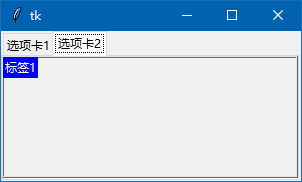1. 加到到2个选项卡里的2个框架都自动扩展到全部选项卡的范围；

2. 加入到2个框架的组件，由于父组件的不同，可以分别用pack()grid()来布局定位，互不干扰。

3. 创建其它组件，只要父组件分别为fr1,fr2，就可以装入更多的组件。…… 其他情况，看上图，以此类推.....丶丌皛

```from tkinter import *
from tkinter.ttk import *

root=Tk()
root.geometry('300x150+888+444')

note1.pack(fill=BOTH,expand=True)

fr1=Frame(root,relief='ridge',borderwidth=1) # 2 创建选项卡1的容器框架
fr2=Frame(root,relief='ridge',borderwidth=1) # 2 创建选项卡2的容器框架

but1=Button(fr1,text='按钮1').pack() # 4 把相关的组件装入对应的框架里
la1=Label(fr2,text='标签1',background='blue',foreground='white').grid()

root.mainloop()```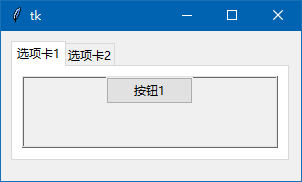```from tkinter import *
from tkinter.ttk import *

root=Tk()
root.geometry('300x150+888+444')

note1=Notebook(root) # 1 创建Notebook组件
note1.pack(fill=BOTH,expand=True)

fr1=Frame(root,relief='ridge',borderwidth=1) # 2 创建选项卡1的容器框架
fr2=Frame(root,relief='ridge',borderwidth=1) # 2 创建选项卡2的容器框架

img1=PhotoImage(file='16-1.png')

but1=Button(fr1,text='按钮1').pack() # 4 把相关的组件装入对应的框架里
la1=Label(fr2,text='标签1',background='blue',foreground='white').grid()

root.mainloop()```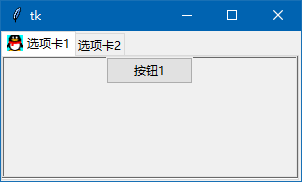```from tkinter import *
from tkinter.ttk import *

root=Tk()
root.geometry('300x150+888+444')

style1 = Style()
style1.configure('my.TNotebook', tabposition='se') # 'se'再改nw,ne,sw,se,w,e,wn,ws,en,es,n,s试试

note1=Notebook(root,style='my.TNotebook') # 1 创建Notebook组件
note1.pack(fill=BOTH,expand=True)

fr1=Frame(root,relief='ridge',borderwidth=1) # 2 创建选项卡1的容器框架
fr2=Frame(root,relief='ridge',borderwidth=1) # 2 创建选项卡2的容器框架

but1=Button(fr1,text='按钮1').pack() # 4 把相关的组件装入对应的框架里
la1=Label(fr2,text='标签1',background='blue',foreground='white').grid()

root.mainloop()```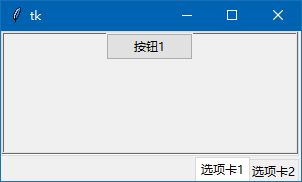tabpostition=’se’ tabpostition=’wn’ 再运行一下，结果：大家自行把tabpostition参数改nw,ne,sw,se,w,e,wn,ws,en,es,n,s试试

Notebook相关方法：

1. hide(TabID)  # 隐藏某选项卡，如 note1.hide(1)   TabID为选项卡序号

2. forget(child)  # 永久删除选项卡

4. select(TabID)  # 选中某选项卡

```from tkinter import *
from tkinter.ttk import *

root=Tk()
root.geometry('350x150+888+444')

note1.pack(fill=BOTH,expand=True)

fr1=Frame(root,relief='ridge',borderwidth=1) # 2 创建选项卡1的容器框架
fr2=Frame(root,relief='ridge',borderwidth=1) # 2 创建选项卡2的容器框架
fr3=Frame(root,relief='ridge',borderwidth=1) # 2 创建选项卡3的容器框架

but1=Button(fr1,text='按钮1').pack() # 4 把相关的组件装入对应的框架里
la1=Label(fr2,text='标签1',background='blue',foreground='white').grid()

fr4=Frame(root,relief='ridge',borderwidth=1) # 准备装入4个按钮
fr4.pack()

note1.select(1) # 选中第2个选项卡
# note1.select(fr2) # 也可以用这种方法，选中第2个选项卡

Button(fr4,text="隐藏",command=lambda:note1.hide(1)).pack(side=LEFT) # 隐藏选项卡2（id=1)
Button(fr4,text="删除",command=lambda:note1.forget(fr3)).pack(side=LEFT)  # 永久删除选项卡3

root.mainloop()```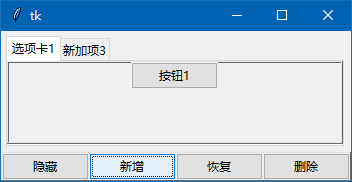1. insert(TabID,child,text)  # 在某个选项卡前插入一个新的选项卡

insert(1, fr3, text=’新插入的选项卡’)

insert(’END’, fr3, text=’最后位置插入选项卡’)

2. tab(TabID, 参数…)   # tab()方法操作选项卡

note1.tab(1,text=’改个名’)  # 为第2个选项卡改名

note1.tab(1,state='disabled')  # 2个选项卡禁止操作

note1.tab(1,state=’normal’)  # 2个选项卡状态正常

note1.tab(1,’text’)  # 返回第2个选项卡的文本

note1.tab(1,’state’)  # 返回第2个选项卡的状态

note1.tab(1)  # 省略第2个参数，就返回所有参数的的字典

3. index(TabID) # 返回选项卡的索引值，如果TabID’end’ 返回选项卡的总数量

index(fr1)  #  返回值为0

index(‘current’)  # 返回鼠标点击处的选项卡的索引值（序号）

<<NotebookTabChanged>>  # 当选项卡发生改变时，此事件发生

```from tkinter import *
from tkinter.ttk import *

root=Tk() # 源码来自wb98.com
root.geometry('350x150+888+444')

note1.pack(fill=BOTH,expand=True)

fr1=Frame(root,relief='ridge',borderwidth=1) # 2 创建选项卡1的容器框架
fr2=Frame(root,relief='ridge',borderwidth=1) # 2 创建选项卡2的容器框架
fr3=Frame(root,relief='ridge',borderwidth=1) # 2 创建选项卡3的容器框架

but1=Button(fr1,text='按钮1').pack() # 4 把相关的组件装入对应的框架里
la1=Label(fr2,text='标签1',background='blue',foreground='white').grid()

note1.select(1) # 选中第2个选项卡
N=note1.index(1) # 得到第2个选项卡的序号
root.title('当前选项卡序号：'+ str(N)) # 用窗体标题来显示当前的选项卡序号

fr4=Frame(root,relief='ridge',borderwidth=1) # 准备装入4个按钮
fr4.pack()

def newk():
note1.insert(1,fr3,text='插入的卡') # 插入新选项卡
note1.select(1) # 选中插入的选项卡

Button(fr4,text="插入",command=newk).pack(side=LEFT) # 插入选项卡（id=1)
Button(fr4,text="改名",command=lambda:note1.tab(1,text='改个名称')).pack(side=LEFT) # 第2个选项卡改名
Button(fr4,text="禁止",command=lambda:note1.tab(1,state='disabled')).pack(side=LEFT)  # 第2个选项卡禁止操作
Button(fr4,text="可用",command=lambda:note1.tab(1,state='normal')).pack(side=LEFT)  # 第2个选项卡恢复正常操作

def selectjob(event):
print('-------------')
root.title('当前选项卡序号：'+ str(note1.index(note1.select()))) # 鼠标点击处的选项卡的序号
print('当前选项卡文本：',note1.tab(note1.select(),'text')) # 返回选中选项卡的文本
print('当前选项卡参数字典：',note1.tab(note1.select())) # 返回选中选项卡的参数字典

note1.bind('<<NotebookTabChanged>>',selectjob) # 选项卡发生变动时触发此事件

root.mainloop()```style1 = Style()

style1.layout('TNotebook.Tab', []) # 隐藏选项卡

```from tkinter import *
from tkinter.ttk import *

root=Tk()
root.geometry('350x150+888+444')

style1 = Style()
style1.layout('TNotebook.Tab', []) # 隐藏选项卡

note1.pack(fill=BOTH,expand=True)

fr1=Frame(root,relief='ridge',borderwidth=1) # 2 创建选项卡1的容器框架
fr2=Frame(root,relief='ridge',borderwidth=1) # 2 创建选项卡2的容器框架

but1=Button(fr1,text='按钮1--选项卡1').pack() # 4 把相关的组件装入对应的框架里
la1=Label(fr2,text='标签1--选项卡2',background='blue',foreground='white').grid()

note1.select(1) # 选中第2个选项卡

fr4=Frame(root,relief='ridge',borderwidth=1) # 准备装入4个按钮
fr4.pack()

Button(fr4,text="选项卡1",command=lambda:note1.select(0)).pack(side=LEFT) # 选中第1个选项卡
Button(fr4,text="选项卡2",command=lambda:note1.select(1)).pack(side=LEFT) # 选中第2个选项卡

root.mainloop()```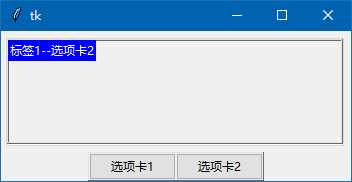```。
style1 = Style()
style1.configure('TNotebook.Tab',font=('宋体',22,'bold'),foreground='red')
# 设置选项卡字体，大小，颜色```

```from tkinter import *
from tkinter.ttk import *

root=Tk() # 源码来自wb98.com
root.geometry('350x150+888+444')

style1 = Style()
style1.configure('TNotebook.Tab',font=('宋体',22,'bold'),foreground='red')
# 设置选项卡字体，大小，颜色

note1.pack(fill=BOTH,expand=True)

fr1=Frame(root,relief='ridge',borderwidth=1) # 2 创建选项卡1的容器框架
fr2=Frame(root,relief='ridge',borderwidth=1) # 2 创建选项卡2的容器框架

but1=Button(fr1,text='按钮1--选项卡1').pack() # 4 把相关的组件装入对应的框架里
la1=Label(fr2,text='标签1--选项卡2',background='blue',foreground='white').grid()

note1.select(1) # 选中第2个选项卡

fr4=Frame(root,relief='ridge',borderwidth=1) # 准备装入4个按钮
fr4.pack()

Button(fr4,text="选项卡1",command=lambda:note1.select(0)).pack(side=LEFT) # 选中第1个选项卡
Button(fr4,text="选项卡2",command=lambda:note1.select(1)).pack(side=LEFT) # 选中第2个选项卡个选项卡恢复正常操作

root.mainloop()```Notebook组件功能还是很强大的，要学的知识很多，我也只是学了一点皮毛，先讲解到这里，以后有机会再就这个组件进行交流。

<< 上一篇 下一篇 >>